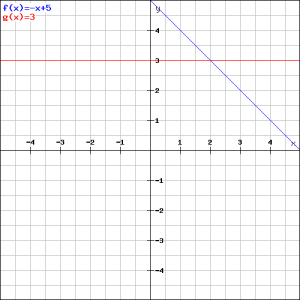# Solution assignment 08 Linear functions and graphs

### Assignment 8

The linegoes through the points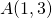and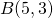and intersects the line: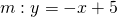in the point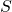.
Calculate the coordinates of.

### Solution

We can use the formula that computes the slope of the linefrom the points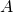and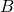, but this is not necessary. We notice that the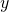-coordinates of both points are equal, meaning that the line is parallel to the-axis and thus has the equation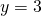. The-coordinate of the intersection pointis: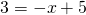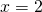and thushas the coordinates, see the figure.